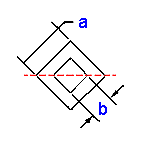Area Moment of Inertia Section Properties Square Tube
Rotated 45 Deg at Center Calculator

Area Moment of Inertia Section Properties Square Tube Rotated 45 Deg at Center Calculator and Equations

This engineering calculator will determine the Area Moment of Inertia Section Properties for the given cross-section. This engineering data is often used in the design of structural beams or structural flexural members. There are two types of section moduli, the elastic section modulus (S) and the plastic section modulus (Z).

 Description Equation Area Moment of Inertia Section Properties = ISection Modulus = Z = I/yRadius of GyrationA = areay = distance from axis to extreme fiber﻿
 Variables Inputs Inch mm Length a (in, mm) = Length b (in, mm) = Calculated Properties Area Moment of Inertia Section I (in4, mm4) = Section Modulus  (in3, mm3) = Radius of Gyration (in3, mm3) = Distance y (in, mm) = Area (Unit2) =Membership Register | LoginHomeEngineering Book StoreEngineering ForumExcel App. DownloadsOnline Books & ManualsEngineering NewsEngineering VideosEngineering CalculatorsEngineering ToolboxGD&T Training Geometric Dimensioning TolerancingDFM DFA TrainingTraining Online EngineeringAdvertising CenterCopyright Notice